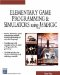# Asin

This command calculates the arcsine. Here is its syntax:

` x = asin(y) `

### Parameters

 x The angle in radians that corresponds to a sine value of y

### Notes

The arcsine can be useful when you perform calculations that involve geometry. For example, the sine of 90 degrees (pi/2 radians) is 1. The arcsine of 1 is then pi/2 (3.1416/2). The arcsine finds the angle in radians that corresponds to a given sine value.

### Sample Code

The following code sends the angle of roll about the y-axis to the screen; this roll is controlled with the left and right arrow keys. The program first finds the angle, then it finds the sine of the angle, and then it uses the asin function to find the angle again based on the sine.

` oworld = New World(); ocamera = New Camera(oworld); sphere = oworld.CreateSphere(50,50,50,25,25); ocamera.MoveBack(1200); sphere.Walk(); //Loop While(TRUE)  {   z = sphere.getyangle();   Y = sin(z);   X = asin(y);   SetText(yangle  + x);    } `Elementary Game Programming & Simulations Using Jamagic (Charles River Media Game Development)
ISBN: 1584502614
EAN: 2147483647
Year: 2002
Pages: 105
Authors: Sergio Perez